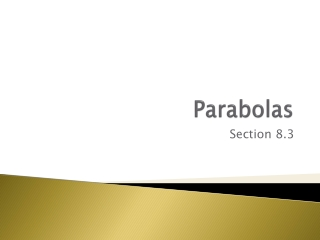DownloadDownload PresentationParabolas

# Parabolas

Télécharger la présentation## Parabolas

- - - - - - - - - - - - - - - - - - - - - - - - - - - E N D - - - - - - - - - - - - - - - - - - - - - - - - - - -
##### Presentation Transcript

1. Parabolas Section 8.3

2. You have studied parabolas in several different lessons, and you have transformed parabolic graphs to model a variety of situations. • Now a locus definition will reveal properties of parabolas that you can use to solve other practical problems.

3. Satellite dishes, used for television, radio, and other communications, are always parabolic. • A satellite dish is set up to aim directly at a satellite. As the satellite transmits signals to a dish, the signals are reflected off the dish surface and toward the receiver, which is located at the focus of the paraboloid. In this way, every signal that hits a parabolic dish can be directed into the receiver.

4. The designs of telescope lenses, spotlights, satellite dishes, and other parabolic reflecting surfaces are based on a remarkable property of parabolas: • A ray that travels parallel to the axis of symmetry will strike the surface of the parabola or paraboloid and reflect toward the focus. • Likewise, when a ray from the focus strikes the curve, it will reflect in a ray that is parallel to the axis of symmetry.

5. A paraboloid is a three-dimensional parabola, formed when a parabola is rotated about its line of symmetry.

6. Definition of a Parabola • A parabola is a locus of points in a plane whose distance from a fixed point called the focus is the same as the distance from a fixed line called the directrix. That is, d1=d2. In the diagram, F is the focus and l is the directrix.

7. A parabola is the set of points for which the distances d1 and d2 are equal. • If the directrix is a horizontal line, the parabola is vertically oriented. • If the directrix is a vertical line, the parabola is horizontally oriented.

8. The directrix can also be neither horizontal nor vertical, creating a parabola that is rotated at an angle.

9. How can you locate the focus of a given parabola? • Suppose the parabola is horizontally oriented, with vertex (0, 0). It has a focus inside the curve at a point, (f, 0). • The vertex is the same distance from the focus as it is from the directrix, so the equation of the directrix is x=-f.

10. You can use this information and the distance formula to find the value of f when the vertex is at the origin.

11. y2=4fx • This result means that the coefficient of the variable x is 4f, where f is the distance from the vertex to the focus. • What do you think it means if f is negative?

12. If the parabola is vertically oriented, the x- and y-coordinates are exchanged, for a final equation of

13. Example Consider the parent equation of a horizontally oriented parabola, y2 = x. • Write the equation of the image of this graph after the following transformations have been performed, in order: a vertical dilation by a factor of 3, a translation right 2 units, and then a translation down 1 unit. Graph the new equation. • Where is the focus of y2 = x? Where is the directrix? • Where is the focus of the transformed parabola? Where is its directrix?

14. a vertical dilation by a factor of 3, a translation right 2 units, and then a translation down 1 unit. • Begin with the parent equation, and perform the specified transformations.

15. Use the standard form, y2 =4fx, to locate the focus and the directrix of y2 = x. • The coefficient of x is 4f in the general form, and 1 in the equation y2 = x. So 4f = 1, or f = 1/4. Recall that f is the distance from the vertex to the focus and from the vertex to the directrix. The vertex is (0, 0), so the focus is (1/4 ,0) and the directrix is the line x =1/4.

16. Transformed Parabola • To locate the focus and the directrix of the transformed parabola, first rewrite the equation as (y +1)2 =9(x -2). • Notice that the coefficient of x in this equation is 9, so 4f =9, or f =2.25. • The focus and the directrix will both be 2.25 units from the vertex in the horizontal direction. • The vertex is (2, 1), so the focus is (4.25, 1) and the directrix is the line x =0.25.

17. InvestigationFold a Parabola • Fold the patty paper parallel to one edge to form the directrix for a parabola. • Mark a point on the larger portion of the paper to serve as the focus for your parabola. • Fold the paper so that the focus lies on the directrix. • Unfold, and then fold again, so that the focus is at another point on the directrix. • Repeat this many times.

18. The creases from these folds should create a parabola. • Lay the patty paper on top of a sheet of graph paper. • Identify the coordinates of the focus and the equation of the directrix, and write an equation for your parabola.